# NCERT Solutions for Class 12 Physics Chapter 6 Electromagnetic Induction

In this chapter, we provide NCERT Solutions for Class 12 Physics Chapter 6 Electromagnetic Induction for English medium students, Which will very helpful for every student in their exams. Students can download the latest NCERT Solutions for Class 12 Physics Chapter 6 Electromagnetic Induction pdf, free NCERT solutions for Class 12 Physics Chapter 6 Electromagnetic Induction book pdf download. Now you will get step by step solution to each question. Class 12 physics is a very important subject for entrance exams like IIT JEE, CPMT, etc.

## NCERT Solutions for Class 12 Physics Chapter 6 Electromagnetic Induction

NCERT Exercises

Question 1.
Predict the direction of induced current in the situations described by the following Figures (a) to (f).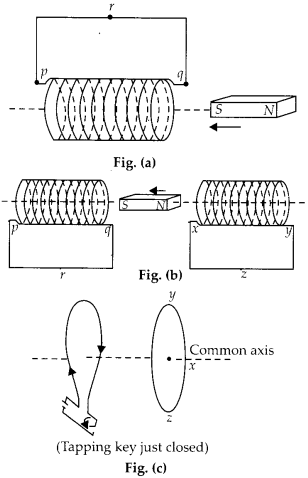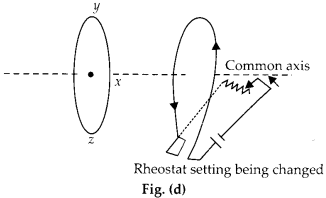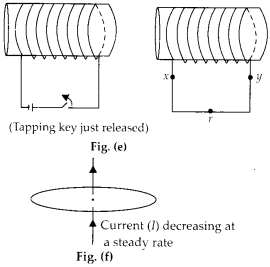Solution:
Direction of induced current in all the situations shown above can be decided in the light of Lenz’s law.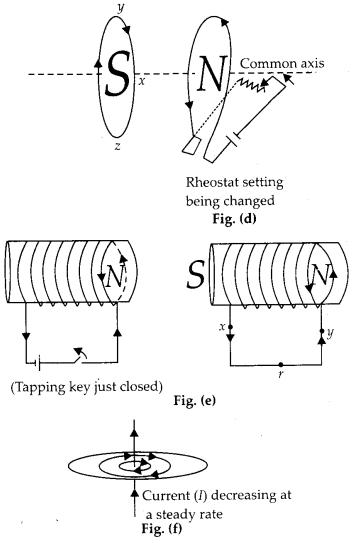Fig. (a) : South pole is moving closer, so the current is clockwise in the end of solenoid closest to magnet.
Fig. (b) : Following Lenz’s law, the current flow anticlockwise in the loop at the left and clockwise in the loop at the right.
Fig. (c) : Inner side of loop-1 become south pole whose strength increasing with increase in current. So the inner side of loop should also become south pole according to Lenz’s law.
Fig. (d) : Current is decreasing with increase in rheostat, so North pole is getting weaker, the current in inner part of loop-1 will flow clockwise.
Fig. (e) : Induced current in the right coil is from X to Y,
Fig. (f) : No induced current since magnetic lines of force are in the plane of the loop.

Question 2.
Use Lenz’s law to determine the direction of induced current in the situations described by figures.
(a) A wire of irregular shape turning into a circular shape:
(b) A circular loop being deformed into a narrow straight wire.Solution:(a) Due to change in shape, area increases and consequently magnetic flux linked with it also increases. Using Lenz’s law, an induced current is set up in the circular wire in the anticlockwise direction to produce opposing flux. So magnetic field due to it is directed upward.
(b) Due to deformation of circular loop into a straight wire, its area decreases and consequently magnetic flux linked with it decreases. So an induced current is set up in the -anticlockwise direction, hence magnetic field is upward.

Question 3.
A long solenoid with 15 turns per cm has a small loop of area 2.0 cm2 placed inside the solenoid normal to its axis. If the current carried by the solenoid changes steadily from 2.0 A to 4.0 A in 0.1 s, what is the induced emf in the loop while the current is changing?
Solution:
When the current changes through the solenoid, a change in magnetic field also take place within it. Initial magnetic field in solenoid,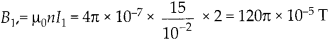Final magnetic field, B2 = μ0nI2

Question 4.
A rectangular wire loop of sides 8 cm and 2 cm with a small cut is moving out of a region of uniform magnetic field of magnitude 0.3 T directed normal to the loop. What is the f e.m.f developed across the cut if velocity of loop is 1 cm s-1 in a direction normal to the (a) longer side (b) shorter side of the loop? For how long does the induced voltage last in , each case?
Solution:
Here A = 2 × 2 = 16 cm2 = 16 × 10 m2, B = 0.3 T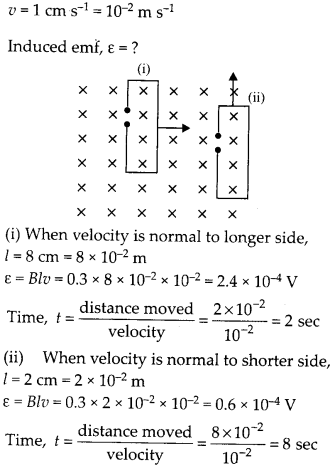Question 5.
A 1.0 m long metallic rod is rotated with an angular frequency of 400 rad s-1 about an axis normal to the rod passing through its one end. The other end of the rod is in contact with a circular metallic ring. A constant and uniform magnetic field of 0.5 T parallel to the axis exists everywhere. Calculate the emf developed between the centre and the ring.
Solution:
Constant and uniform magnetic field is parallel to axis of the wheel and thus normal to plane of the wheel.Question 6.
A circular coil of radius 8.0 cm and 20 turns is rotated about its vertical diameter with an angular speed of 50 rad s-1 in a uniform horizontal magnetic field of magnitude 3.0 × 10-2 T. Obtain the maximum and average emf induced in the coil. If the coil forms a closed loop of resistance 10 ohm, calculate the maximum value of current in the coil. Calculate the average power loss due to Joule heating. Where does this power come,from?
Solution:
If the circular coil rotates in the magnetic field B at an angular velocity ot, then instantaneous induced emf can be calculated.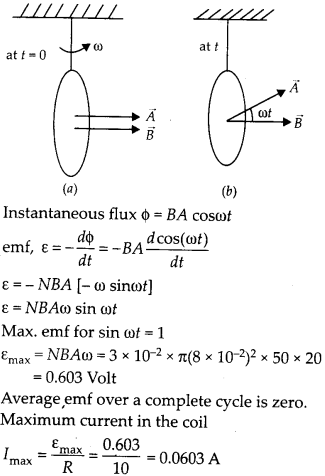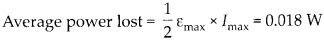Source of the power is work done in rotating the coil.

Question 7.
A horizontal straight wire 10 m long extending from east to west is falling with a speed of 5.0 m s1, at right angles to the horizontal component of the earth’s magnetic field, 0.30 × 10-4 Wb m-2.
(a) What is the instantaneous value of the emf induced in the wire?
(b) What is the direction of the emf?
(c) Which end of the wire is at the higher electrical potential?
Solution:
The direction of earth’s magnetic field is in the direction of geographical south to geographical northLet us take a convenient way to represent all the directions.(a) Instantaneous emf ε = Bυl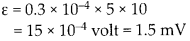(b) Direction of emf. will be west to east.
(c) West end of the wire will be charged at higher potential.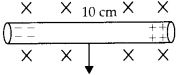Question 8.
Current in circuit falls from 5.0 A to 0.0 A in 0.1 s. If an average emf of 200 V induced, give an estimate of the self-inductance of the circuit.
Solution:
Let ‘L’ is the coefficient of self inductance, the back emf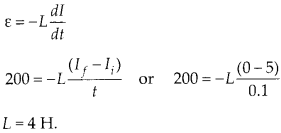Question 9.
A pair of adjacent coils has a mutual inductance of 1.5 H. If the current in one coil changes from 0 to 20 A in 0.5 s, what is the change of flux linkage with the other coil?
Solution:
Let the current changes from 0 to 20 A in coil 1 and we are looking for change of flux linked with coil 2.Question 10.
A jet plane is travelling towards west at a speed of 1800 km h-1. What is the voltage difference developed between the ends of the wing having a span of 25 m, if the Earth’s magnetic field at the location has a magnitude of 5 × 10-4 T and the dip angle is 30°.
Solution:
Earth magnetic field will have two components, BH and Bv. It is vertical component which develop induced emf across the wing in N-S direction.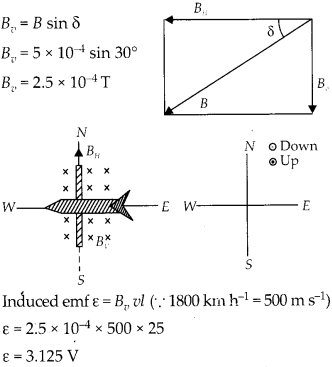Question 11.
Suppose the loop shown in figure is stationary but the current feeding the electromagnet that produces the magnetic field is gradually ? reduced so that field decreases from its initial value of 0.3 T at the rate of 0.02 T s-1. If the cut is joined and the loop has a resistance of 1.6Ω, how much power is dissipated by the loop as heat? What is the source of this power?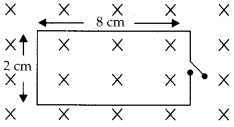Solution:
Here area is constant but the magnetic field is reducing at a constant rate.Source of the power is work done in changing magnetic field.

Question 12.
A square loop of side 12 cm with its sides ‘r parallel to X and Y axes is moved with a velocity of 8 cm s’1 in the positive x-direction in an environment containing a magnetic field in the positive z-direction. The field is neither uniform in space nor constant in time. It has a gradient of 10-3 T cm-1 along the negative x:direction (that is it increases by 10“3 T cm-1 as one moves in the negative x-direction) and it is decreasing in time at the rate of 10-3 T s–1. r Determine the direction and magnitude of the induced current in the loop if its resistance is 4.50 mΩ
Solution:

Each side of square loop is 12 cm and magnetic field is decreasing along x direction.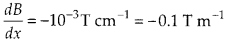Also the magnetic field is decreasing with time at constant rate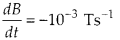Induced emf and rate of change of magnetic flux due to only time variation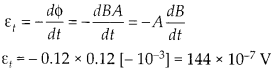Induced emf and rate of change of magnetic flux due to change in position.Both the induced emf have same sign and thus adds to provide net Induced emf in the loop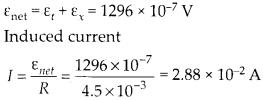Question 13.
It is desired to measure the magnitude of field between the poles of a powerful loud speaker magnet. A small flat search coil of area 2 cm2 with 25 closely wound turns, is positioned normal to the field direction, and then quickly snatched out of field region. Equivalently, one can give it quick 90° turn to bring its plane parallel to the field direction. The total charge flown in the coil (measured by a ballistic galvanometer connected to coil) is 7.5 mC. The combined resistance of coil and the galvanometer is 0.5 Ω. Estimate the field strength of magnet.
Solution:
Let the magnetic field between poles of loud speaker magnet is B.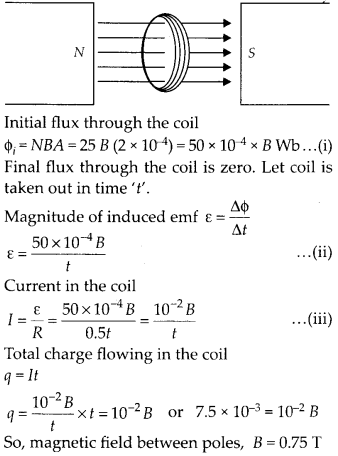Question 14.
Figure shows a metal rod PQ resting on the smooth rails AB and positioned between the poles of a permanent magnet. The rails, the rod, and the magnetic field are in three mutual perpendicular directions. A galvanometer G connects the rails through a switch K. Length of the rod = 15 cm, B = 0.50 T, resistance of the closed loop containing the rod = 9.0 mQ. Assume the field to be uniform.
(a) Suppose K is open and the rod is moved with a speed of 12 cm s-1 in the direction shown. Give the polarity and magnitude of the induced emf.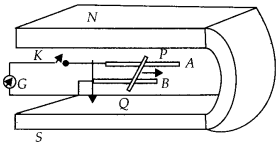(b) Is there an excess charges built up at the ends of the rods when K is open? What if K is closed?
(c) With K open and the rod moving uniformly, there is no net force on the electrons in the rod PQ even though they do experience magnetic force due to the motion of the rod. Explain
(d) What is the retarding force on the rod when If is closed?
(e) How much power is required (by an external agent) to keep the rod moving at the same speed (= 12 cm s-1) when K is closed? How much power is required when K is open?
(f) How much power is dissipated as heat in the closed circuit? What is the source of this power?
(g) What is the induced emf in the moving rod if the magnetic field is parallel to the rails instead of being perpendicular?
Solution:
Here rails, rod and magnetic field are in three mutually perpendicular directions.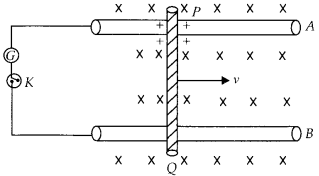(a) Switch K is open and rod moves with speed of 12 cm-1 three mutually perpendicular directions. Induced emf/motional emf
ε = Bυl ε = 0.5 × 12 × 10-2 × 15 × 10-2 = 9 mV
(b) When the K is open, upper end of the rod become positively charge, and lower end become negatively charged.
When the K is closed the charge flows in closed circuit but the excess charge is maintained by the flow of charge in the moving rod under magnetic force.
(c) In the state when K is open very soon a stage is reached when force due to electric field which is due to potential difference induced balances the magnetic force on electrons. eE = Beυ$e\frac { V }{ l } =Be\upsilon$ Motional emf V = Bυl.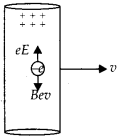(d) When the key is closed the current flows in a loop and the current carrying wire experience a retarding force in the magnetic field.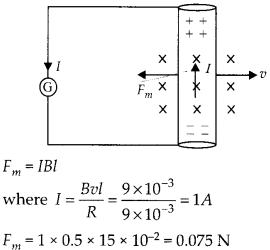(e) To keep the rod moving in closed circuit at constant speed the force required iswhen key K is open, no current flows and hence no retarding force, so no power is required to move at constant speed.
(f) Power lost in closed circuit due to flow of current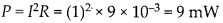Power provided by external force to move the rod at constant speed is the source of this power lost.
(g) If B is parallel to rails, the induced/ motional emf will be zero.

Question 15.
An air cored solenoid with length 30 cm, area of cross-section 25 cm2 and number of turns 500, carries a current of 2.5 A. The current is suddenly switched off in a brief time of 10-3 s. How much is the average back emf induced across the ends of the open switch in the circuit? Ignore the variation in magnetic field near the ends of the solenoid.
Solution:
Magnetic field inside solenoid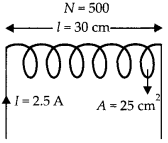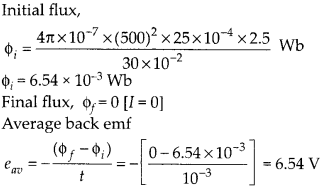Question 16.
(a) Obtain an expression for mutualin ductance , between a long straight wire and a square loop of side ‘a’ as shown in figure.(b) Now assume that straight wire carries a current of 50 A and the loop is moved to the right with a constant velocity, v = 10 m s-1. Calculate the induced emf in the loop at the instant when x = 0.2 m. Take a = 0.1m and assume that loop has a large resistance.
Solution:
(a) As the magnetic field will be variable with distance from long straight wire, so the flux through square loop can be calculated by integration.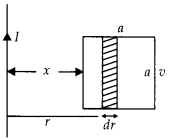Let us assume a width ‘dr’ of the square loop at a distance ‘r’ from straight wire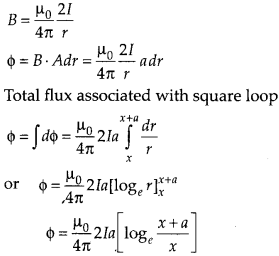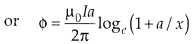(b) The square loop is moving right with a constant speed v, the instantaneous flux can be taken as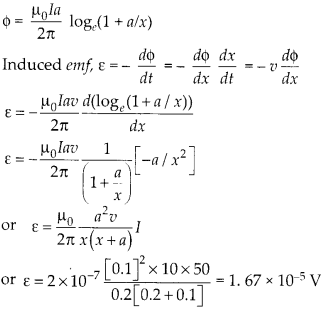Question 17.
A line charge λ per unit length is lodged uniformly onto the rim of a wheel of mass M and radius R. The wheel has light non-conducting spokes and is free to rotate without friction about its axis. A uniform magnetic field extends over a circular region within the rim.$\overrightarrow { B } =-B_{ 0 }\hat { k } (\underline {  It is given by = o (otherwise) What is the angular velocity of the wheel after the field is suddenly switched off?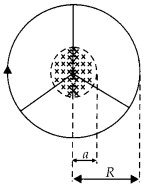Solution:
According to Faraday’s law of electromagnetic induction the induced emf isThus a relation between electric field and rate of change of flux can be established,E exist along circumference of radius ‘a’ due to change in magnetic flux.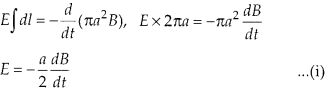Linear charge density on rim is A. So, total charge on rim Q = λ2πa …(ii) Electric Force on the charge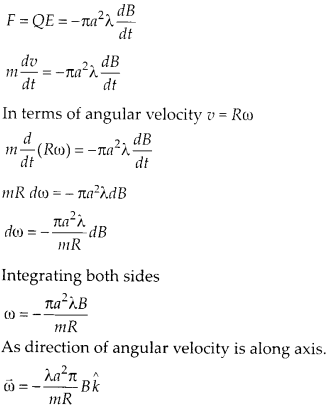All Chapter NCERT Solutions For Class12 Physics

—————————————————————————–

All Subject NCERT Solutions For Class12

*************************************************

Remark:

I think you got complete solutions for this chapter. If You have any queries regarding this chapter, please comment on the below section our subject teacher will answer you. We tried our best to give complete solutions so you got good marks in your exam.

If these solutions have helped you, you can also share Careerkundali.in to your friends.

Best of Luck!!